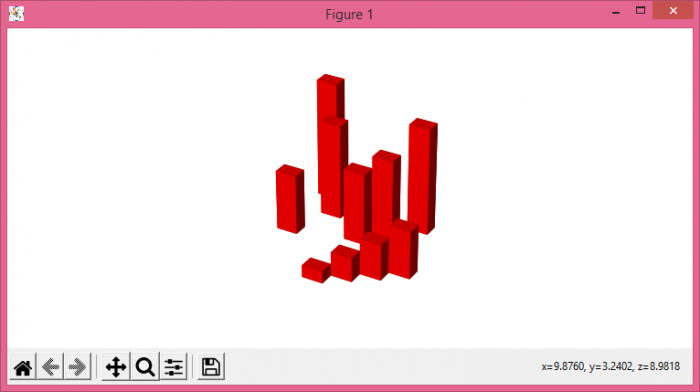# Plot 3D bars without axes in Matplotlib

To plot 3D bars without axes, we can take the following steps

• Set the figure size and adjust the padding between and around the subplots.
• Create a new figure or activate an existing figure using figure() method.
• Add an axes to the cureent figure as a subplot arrangement.
• Create x3, y3 and z3 data points using numpy.
• Create dx, dy and dz data points using numpy.
• Use bar3d() method to plot 3D bars.
• To hide the axes, use axis('off') class by name.
• To display the figure, use show() method.

## Example

import numpy as np
from matplotlib import pyplot as plt
plt.rcParams["figure.figsize"] = [7.50, 3.50]
plt.rcParams["figure.autolayout"] = True
fig = plt.figure()
x3 = [1, 2, 3, 4, 5, 6, 7, 8, 9, 10]
y3 = [5, 6, 7, 8, 2, 5, 6, 3, 7, 2]
z3 = np.zeros(10)
dx = np.ones(10)
dy = np.ones(10)
dz = [1, 2, 3, 4, 5, 6, 7, 8, 9, 10]
ax1.bar3d(y3, x3, z3, dx, dy, dz, color="red")
ax1.axis('off')
plt.show()

## Output